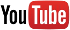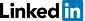Terms of serviceBrokking .net Let's keep it simpleHome
TL-blog
 DIY multicopter / RPAS flight controller          YMFC-32 - The STM32 Arduino quadcopter          YMFC-32 - The STM32 Arduino autonomous quadcopter          YMFC-AL - The self-leveling Arduino quadcopter          YABR - The Arduino Balancing Robot          Building an electric skateboard          Automated reflow hot plate          Building the Stepcraft 420
ProjectsArticlesDonationBrokking.net - Video: YMFC-32| Compass implementation| The STM32 - Arduino DIY drone

# YMFC-32| Compass implementation| The STM32 - Arduino DIY drone

This page contains the full script that I used for making this video.

Hello and welcome back to the YMFC-32 quadcopter video series. In this video I will explain how I implemented the compass in the YMFC-32 code.

Implementing a compass is necessary so the quadcopter knows what direction is north. With this information it's possible to add features like head-lock and GPS-hold.

But first we need to know something about the hardware. An electronic compass is not the same as a normal compass as shown here. The electronic compass that I'm using for the YMFC-32 is the commonly used HMC5883L from Honeywell. This compass is also used in most GPS modules for the Ardupilot.

Inside this tiny sensor there are 3 magneto resistive sensors that can detect the Earth's magnetic field and its magnitude. And with the correct calculations we can calculate the direction of the magnetic field relative to the sensor.

And basically that's all there is to know about the sensor. Now let's have a look at what the sensor is measuring.

As most of you already know the Earth's magnetic field can be visualized with an image like this. And based on this image we can already spot a few challenges.

Here you can see that we have a magnetic pole and a geographic pole. The magnetic pole is given by the earth's magnetic field. The geographic pole is used for the GPS coordinates. And yes, there is a difference between them.

And to make things worse this difference can vary per location. Luckily there are maps like these available that will show you the difference between the magnetic pole and the geographic pole. You can find a link to these maps in the description.

I live in the Netherlands and the difference between the magnetic and geographic pole is near zero. But there are regions on the earth where you really need to take this difference into account. If you ignore this the quadcopter cannot hold its GPS position because its correcting in the wrong direction.

Next thing on the challenge list are these magnetic field lines. Over here they run almost parallel to the earth's surface. But here they are pointed towards the earth. This is called inclination. And again there is a map available where the inclination is given for the different regions.

If I place my tree axis compass parallel to the earth's surface you can see that with a low inclination there is almost no reading from the Z-axis.

But with a high inclination the value of the Z-axis is also increasing. This shows us that not only the X and Y-axis need to be part of the calculations but also the Z-axis.

Same is true when the quadcopter is tilting around its roll and pitch axis. When the inclination is 30 degrees and the quadcopter is also tilting 30 degrees you can see that only the Z-axis is detecting the earth's magnetic field. So the pitch and roll angles of the quadcopter are also needed for the calculation of the quadcopter heading.

Another thing that we need to take into account is interference of the magnetic field around the sensor. When I place the compass on my desk I can print the raw compass value of one of the axis.

Now look what happens when I approach the compass with a large brushed motor. You can see that the raw value changes significant. However, if I now take a brushless motor you can see that there is a minimum change. And that of course is a good thing to know.

Here I have another test setup. In this test I will run 5amps through a wire that is running in close proximity of the compass.

As you can see high current wiring will also interfere with the compass. Normal hover currents with the YMFC-32 can run up to 15 amps without any problems.

In short, that is one of the reasons why GPS modules with integrated compass modules are placed away from motors and high current wiring.

However, on ready to fly models you can program the behavior of the individual motor currents into the compass calculations. This way it's possible to reduce the interference inside the drone. But this technique will not be used in the YMFC-32 code as it makes the code unnecessarily complex.

As you can see I placed the compass on a stick above the quadcopter. This way the interference from the motors and electrical system is minimal.

And with this knowledge we can start building the code that will calculate the heading of the quadcopter.

Before we dive in I want to show you these two papers that explain the implementation of the compass in detail. So if you have any questions left after watching this video please read these papers first. A link to these papers is in the description.

Ok, calculating the heading is relatively easy. Here I have simulated the X and Y-axis of the compass. If both X and Y-axis measure the same value the angle can be calculated with the inverse tangents function as shown here.

So this angle is 45 degrees. Meaning that the magnetic heading is 360 minus 45 is 315 degrees. To get the geographic heading we need to subtract the value as shown on this map. For example, in New York we need to subtract negative 12 degrees from the magnetic heading.

In total, the geographic heading is 327 degrees.

No problems so far. Ok, back to the test model. As explained, if I tilt the compass the value of the axis will change. This is because the inclination of the field lines through the compass change.

To overcome this problem we have to keep the compass virtually level with the pitch and roll axis of the quadcopter. When doing this we also need the Z-axis of the compass as it also detects the earth's magnetic field.

Luckily we don't have to figure out the mathematical formula as it is already given in the documents.

For the X-axis we will use this formula and for the Y-axis we will use this formula.

In the Arduino code these functions look like this. Please note that the sine, cosine and tangent functions use radians instead of degrees. That is why we need to multiply the angle by phi divided by 180.

The minus signs are used to invert the angle direction.

Now that the compass is virtually level we can calculate the magnetic north. And that is all done in this part.

Last thing that we need to do is to subtract the declination to get the geographic north.

And that's it, we now have the geographic heading of the quadcopter.

To get an accurate, fast reacting and stable heading we will also use the gyro yaw sensor as a base. This will be the same method as with the pitch and roll angle.

Every loop the traveled yaw angle is calculated and added to the angle_yaw variable. Because it's in degrees we need to make sure that it stays between 0 and 360 degrees.

This part will calculate the difference between the gyro yaw heading and the compass heading and execute a small correction to compensate for the gyro's drift.

And with that we get a very fast reacting compass heading.

Ok, very important. To get the best results it's important to calibrate the compass. The goals is that after calibration all the compass axis will output the same value when the axis is pointing north.

When there is no interference and with all the offsets set to zero the output of the X and Y-axis will look like this circle.

However, due to interference on the quadcopter and a shifted zero point the circle might look like this. The zero point is shifted and the minimum and maximum values of the X and Y-axis are not the same. This will result in an inaccurate heading.

The fix is very simple, we need to calibrate the compass. The compass calibration method of the YMFC-32 is very simple. Only after uploading the YMFC-32 flight controller code or after hardware modifications you need to perform a compass calibration. To do this you need to take the quadcopter to a field where no magnetic sources are present.

First you need to know where the magnetic north is. After that, move both sticks to the top right corner. The red led will indicate that the calibration is in progress.

Now rotate the quadcopter around the pitch axis in the direction of the magnetic north. After a minimum of two rotations you can switch to the roll axis and again make at least two rotations.

Keep the quadcopter away from the transmitter and move the stick in the nose up direction. The red led goes off and the other led will flash fast. This means that the values are stored in the STM32.

During calibration the minimum and maximum value of every axis are registered by the flight controller program. With these values it's possible to compensate for any distortion and produce a nice and centered circle like this on.

At startup these values will be used to calculate the zero offset and the scale to compensate the compass readings.

After calibration you can check the workings of the compass with the telemetry receiver.

And that is how the compass function of the YMFC-32 works. I hope that you learned something new and if so please give this video a thumbs up.

Thank you for watching and see you next time.